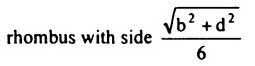Search

# Strength of Materials(121-140)

Updated: Apr 28, 2020

121. A short column of external diameter of 250 mm and internal diameter of 150 mm carries an eccentric load of 1000 kN. The greatest eccentricity which the load can have without producing tension anywhere is 42.5

122. The core of a rectangular section with sides b and d is a123. A reinforced cement concrete footing as shown in Fig. 10.9 carries a concentrated load at P so as to produce maximumbending stresses due to eccentricities about x-x axis and y-y axis as 100 kN/m²

and 150 kN/m² respectively. If the direct stress due to loading is 175 kN/m² (com

pressive), then the intensity of resultant stress at corner B will be

125 kN/m² compressive

124. The ratio of intensity of stress in case of a suddenly applied load to that in case of a gradually applied load is 2

125. Proof resilience is the maximum energy stored at elastic limit

126. Strain energy stored in a member is given by 0.5 x stress x strain x volume

127. A rectangular block of size 200 mm x 100 mm x 50 mm is subjected to a shear stress of 100 N/mm². If modulus of rigidity of material is 1 x 105 N/mm², strain energy stored will be 50 Nm

128.A steel rod of cross sectional area equal to 1000 mm² is 5 m long. If a pull of 100 kN is suddenly applied to it, then the maximum stress intensity will be 200N/mm²

129.Two circular bars A and B of same material and same length are of diameters

DA and DB respectively. The bars are subjected to the same torque T. The ratio

of strain energies stored in the bars A and B is proportional to130.Two identical bars, one simply supported and other fixed at ends, are acted upon by equal loads applied at the midpoints. The ratio of strain energy stored in the simply supported beam and the fixed ended beam is 4

131.If the depth of a beam of rectangular section is reduced to half, strain energy

stored in the beam becomes 8 times

132.The system which is shown in Fig consists of two prismatic bars of same

length L and same axial rigidity AEThe system lies in the vertical plane and carries a vertical load P at joint X, The

vertical deflection of joint X is 2PL/AE

133.The specimen in a Charpy impact test is supported as a simply supported beam

134.Impact test enables one to estimate the property of toughness

135.The phenomenon of decreased resistance of a material to reversal of stress is called fatigue

136.The property of metal which allows it to deform continuously at slow rate without any further increase in stress is known as creep

137. The stress below which a material has a high probability of not failing under

reversal of stress is known as endurance limit

138. A three hinged parabolic arch rib is acted upon by a single load at the left quarter point. If the central rise is increased and the shape of arch altered to segmental without changing the other details, the horizontal thrust will

decrease definitely

139. For ductile materials, the most appropriate failure theory is

maximum shear stress theory

140. At a point in a steel member, the major principal stress is 2000 kg/cm² and the minor principal stress is compressive. If the uni-axial tensile yield stress is 2500

kg/cm², then the magnitude of the minor principal stress at which yielding will

commence, according to the maximum shearing stress theory, is 500 kg/cm²